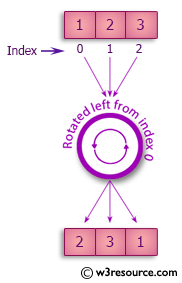﻿ Swift Array Programming Exercise: Rotate the elements of an array of integers to left direction - w3resource# Swift Array Programming Exercises: Rotate the elements of an array of integers to left direction

## Swift Array Programming: Exercise-5 with Solution

Write a Swift program to rotate the elements of an array of integers to left direction. Therefore {1, 2, 3} yields {2, 3, 1}.

Pictorial Presentation:Sample Solution:

Swift Code:

``````func rotate_left_3(_ arra: [Int]) -> [Int] {
var new_arra = arra
new_arra.removeFirst()
new_arra.append(arra.first!)
return new_arra
}

print(rotate_left_3([1, 2, 3]))
print(rotate_left_3([-1, 0, 1]))
print(rotate_left_3([0, 10, 0]))
```
```

Sample Output:

```[2, 3, 1]
[0, 1, -1]
[10, 0, 0]
```

Swift Programming Code Editor:

Improve this sample solution and post your code through Disqus

What is the difficulty level of this exercise?

﻿